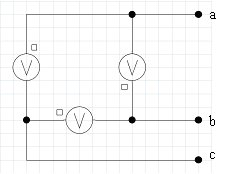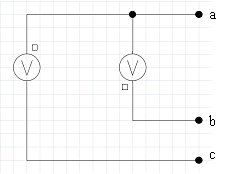# Triangle-connected three-phase voltage source

## Δ - connected three-phase voltage source

The analysis methods for describing the equation corresponding to the electrical circuit (mesh current method in Flux 2D and method of the node integrated potentials in Flux 3D) does not allows the representation of a Δ - connected three-phase voltage source by its real schematic.

## Overcoming the problem

This problem can be overcome by removing one voltage source (see figure below). This will impose the correct potentials at the points a, b and c.

In 2D, the currents through the two sources are false, but the power balance is correct.

The real schematic and the used Flux model are given in the figure below.

real schematic: do not use in Flux

Flux model of schematic: use this